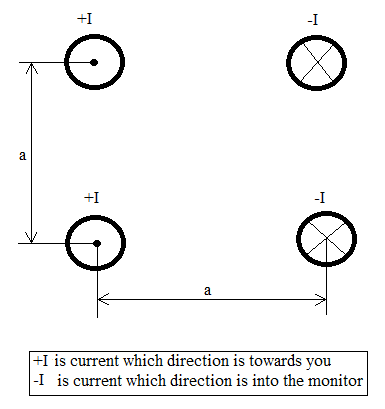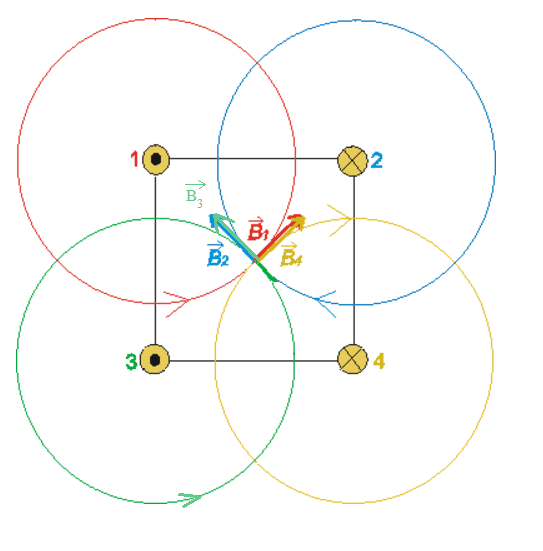# The size and direction of magnetic induction and force

## Homework Statement

Hello, I have this problem:
Four long straight parallel wires are placed in vacuum according to the picture, the distance between each wire is a = 20 cm. Through each wire flows electric current I = 20 A in the specified direction. What is the size and direction of magnetic induction in the middle of this system? What is the size and direction of the force acting on one meter of the bottom left wire?

-

## The Attempt at a SolutionI drew the line of forces following the Ampere's right hand law, and also the vectors of magnetic inducion of each wire:now my answer is how do I compute the size of the resulting induction, and my second question is how do I find out the force acting on 1 meter of the lower left wire?

andrewkirk
Homework Helper
Gold Member
Do they really want to know the strength of magnetic induction? Won't that be zero, because induction arises from the time variation of mag fields and this system is steady state? The vectors you have drawn are of mag fields ##\vec B##, not of induction.

If it's just the mag field that's wanted, not induction, then the Biot-Savart law will do the job.

Do they really want to know the strength of magnetic induction? Won't that be zero, because induction arises from the time variation of mag fields and this system is steady state? The vectors you have drawn are of mag fields ##\vec B##, not of induction.

If it's just the mag field that's wanted, not induction, then the Biot-Savart law will do the job.

Yes they want the strength of magnetic induction, if it is zero, then fine I will write it as a solution. Now can you please give me a hint with identifying the direction and strength of the force acting on one meter of left bottom wire, please?

andrewkirk